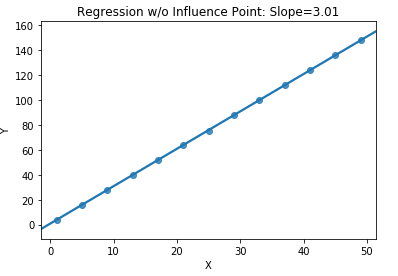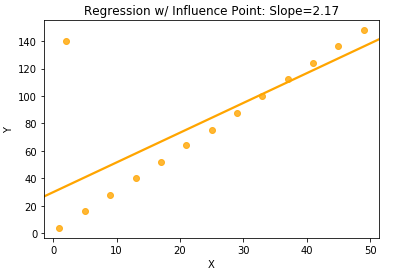# What is a high influence point?

High influence points are observations that most influence, hence the name, the shape of the regression equation. If an observation is a high influence point, the slope of the regression line would change significantly if that one point was removed from the data set. While outliers and leverage points are defined in the context of the target and feature space, respectively, influence points are found in the (X,Y) space, as for an observation to have a large sway over the regression equation, it requires information from both the X and Y spaces.

In the case of a single predictor, a high influence point would be one that either bends the line noticeably upward or downward, thus having an undue effect on the estimate of the slope. The effect of an influence point is shown in the dummy data below, where the presence of a single observation reduces the magnitude of the slope of the regression by almost ⅓. However, in the third graph, an observation that is both a leverage point (in X) and outlier (in Y) is not a high influence point because it has little effect on the slope of the regression despite being isolated in both the X and Y space.The most common diagnostic for detecting influence points is Cook’s Distance, and points that have large Cook values might need to be investigated further. In the process of data exploration, it is important to verify the quality of the data being captured, as it is often the case that these points are due to issues in the underlying collection of the data.

#### How can qualitative predictors be incorporated in linear regression?

Find out all the ways
that you can